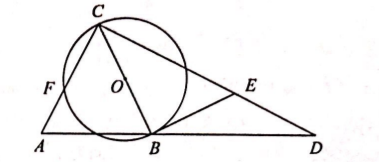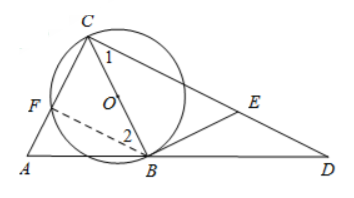(1) 求证: $B E$ 为 $\odot O$ 的切线:
(2) 若 $A F=2, \tan A=2$, 求 $B E$ 的长.【答案】 (1) 证明: $\because A C=B C, E B=E D$
$\therefore \angle A=\angle A B C, \angle D=\angle E B D$
$\because C D \perp A C$
$\therefore \angle A+\angle D=90^{\circ}$
$\therefore \angle A B C+\angle E B D=90^{\circ}$
$\therefore \angle C B E=90^{\circ}$
$\because B C$ 是 $\odot O$ 的直径.
$\therefore B E$ 是 $\odot O$ 的切线

（2）$\because B C$ 是 $\odot O$ 的直径
$\therefore \angle B F C=\angle B F A=90^{\circ}$

\begin{aligned} & (x+2)^2=x^2+4^2 \quad \therefore x=3 \quad \therefore C F=3, B C=5 . \\ & \because \angle A C B=\angle A F B=90^{\circ} \therefore B F // C D \\ & \therefore \angle 1=\angle 2 \\ & \text { 又 } \because \angle C F B=\angle E B C=90^{\circ} \\ & \therefore \triangle C F B C \triangle E B C \\ & \therefore \frac{F C}{B E}=\frac{F B}{B C} \end{aligned}

$\therefore \frac{3}{B E}=\frac{4}{5} \quad \therefore B E=\frac{15}{4}$